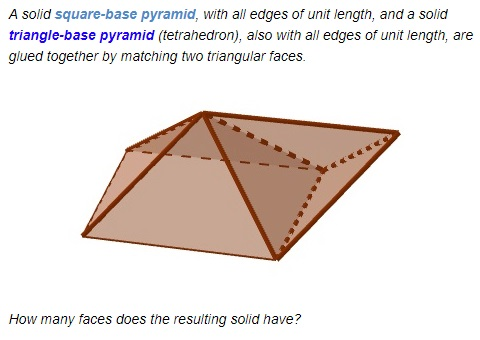# Three Pyramids are Better Than Two

The applet presents a tool for investigating an infamous problem. It showed up in 1980 as a practice problem on the Preliminary Scholastic Aptitude Exam, but, to an embarrassment of the Educational Testing Service, the marked answer was incorrect.

The problem is this [Winkler, p. 43]:No try playing with the GeoGebra applet below:

The expected solution was this. A square pyramid has 5 faces and a tetrahedron 4 faces. When two triangular faces are eliminated by gluing them together there remain 5 + 4 - 2 = 7 faces. By adding a second square-base pyramid, it becomes absolutely clear that, in the problem, there are two pairs of adjacent faces that align on a single plane (i.e., are coplanar) so that the resulting solid has two faces less than expected: 5 in all.

(As an aside, what is the relationship between the volumes of the square pyramid and the tertrahedron? Find out.

### References

1. P. Winkler, Mathematical Puzzles: A Connoisseur's Collection, A K Peters, 2004• Right Pentagonal Prism
• Square Pyramid
• Right Triangular Prism
• Twisted Triangular Prism
• Tetrahedron: an Interactive Model
• Octahedron: an Interactive Model
• Cube: an Interactive Model
• Icosahedron: an Interactive Model
• Dodecahedron: an Interactive Model
• Cube In Octahedron
• Octahedron In Cube
• Octahedron In Tetrahedron
• Tetrahedron In Cube
• Icosahedron In Cube
• Great Stellated Dodecahedron
• Lennes' Polyhedron
• Triangulated Dinosaur
• Volumes of Two Pyramids
• Császár Polyhedron 1
• Császár Polyhedron 4
• Szilassi Polyhedron
• Dissection of a Square Pyramid
•